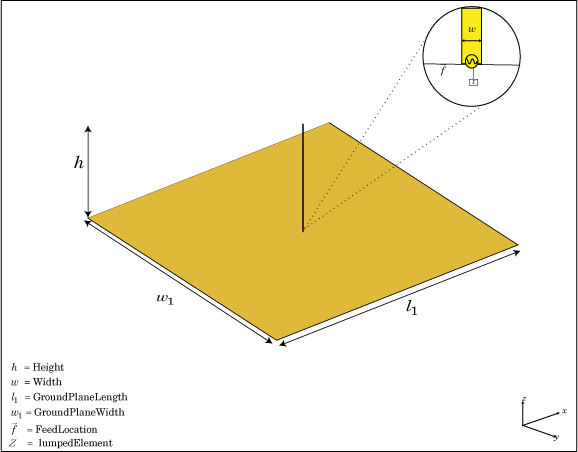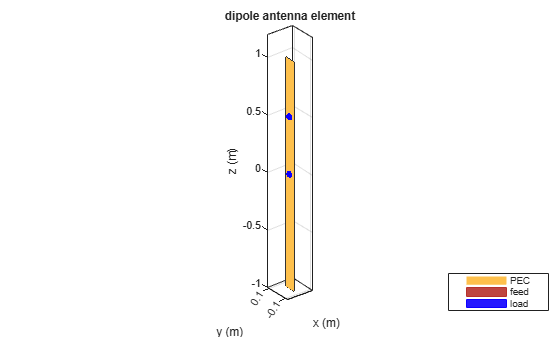Documentation

lumpedElement

Lumped element circuit to load antenna

Description

le = lumpedElement creates a lumped element circuit. The default value is an empty lumpedElement object.When you load an antenna using a lumped resistor, capacitor, or inductor, the electrical properties of the antennas changes. These lumped elements are typically added to the antenna feed. You can use lumped elements to increase the bandwidth of the antenna without increasing the size of the antenna.

example

le = lumpedElement(Name,Value) returns the lumped element circuit based on the properties specified by one or more Name,Value pair arguments.

Examples

collapse all

Create a resistor with 50 Ohms of impedance. Any pure resistive load has a nonvariable impedance when the frequency changes.

le = lumpedElement('Impedance',50);

Create a dipole antenna. Calculate the impedance of the antenna without loading the antenna.

d = dipole;
i1 = impedance(d,70e6)
i1 = 72.9381 - 1.2090i

Load the antenna using a frequency-independent resistor. Calculate the impedance of the antenna.

i1e1 = impedance(d,70e6)
i1e1 = 1.2294e+02 - 1.2090e+00i

Change the frequency to 85 MHz and calculate the impedance of the antenna.

ile2 = impedance(d,85e6)
ile2 = 2.3009e+02 + 1.1005e+02i

Create a dipole antenna using one load at the antenna feed and one load at a location above the antenna feed.

Create a dipole antenna.

d = dipole;

Create two lumped elements to load the dipole antenna.

One lumped element of impedance, 50 Ohms, loads the antenna at the feed.

l1 = lumpedElement('Impedance', complex(50, -20), 'Location', 'feed');

The second lumped element of complex impedance, 50+ j*20 Ohms, loads the antenna at the top. Locate the load half distance from the feed.

l2 = lumpedElement('Impedance', complex(50, -20), 'Location', [0 0 0.5]);

Add the two loads to the dipole antenna.

d.Load = [l1, l2];

View the dipole antenna.

show(d);Input Arguments

collapse all

Name-Value Pair Arguments

Specify optional comma-separated pairs of Name,Value arguments. Name is the argument name and Value is the corresponding value. Name must appear inside quotes. You can specify several name and value pair arguments in any order as Name1,Value1,...,NameN,ValueN.

Example: 'Frequency',2e9

Complex impedance of circuit, specified as the comma-separated pair consisting of 'Impedance' and a real or complex vector of z-parameters in ohms.

Example: 'Impedance',complex(75,30) specifies a complex impedance of 75+i30.

Data Types: double

Frequency of operation, specified as the comma-separated pair consisting of 'Frequency' and a real vector in Hz.

Example: 'Frequency',[10e6,20e6,30e6]

Data Types: double

Location of load, specified as the comma-separated pair consisting of 'Location' and Cartesian coordinates.

Example: 'Location',[0 0 0.5]

Data Types: double

Output Arguments

collapse all

Lumped element, returned as a lumpedElement object. The real part of the complex number indicates the resistance. The imaginary part of the complex number indicates the reactance.